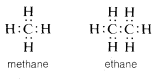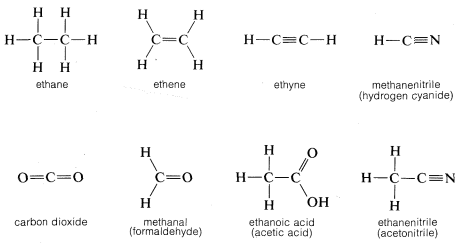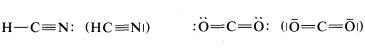# 2.1: Structural Formulas

The building block of structural organic chemistry is the tetravalent carbon atom. With few exceptions, carbon compounds can be formulated with four covalent bonds to each carbon, regardless of whether the combination is with carbon or some other element. The two-electron bond, which is illustrated by the carbon-hydrogen bonds in methane or ethane and the carbon-carbon bond in ethane, is called a single bond. In these and many related substances, each carbon is attached to four other atoms:There exist, however, compounds such as ethene (ethylene), $$C_2H_4$$, in which two electrons from each of the carbon atoms are mutually shared, thereby producing two two-electron bonds, an arrangement which is called a double bond. Each carbon in ethene is attached to only three other atoms:Similarly, in ethyne (acetylene), $$C_2H_2$$, three electrons from each carbon atom are mutually shared, producing three two-electron bonds, called a triple bond, in which each carbon is attached to only two other atoms:Of course, in all cases each carbon has a full octet of electrons. Carbon also forms double and triple bonds with several other elements that can exhibit a covalence of two or three. The carbon-oxygen (or carbonyl) double bond appears in carbon dioxide and many important organic compounds such as methanal (formaldehyde) and ethanoic acid (acetic acid). Similarly, a carbon-nitrogen triple bond appears in methanenitrile (hydrogen cyanide) and ethanenitrile (acetonitrile).By convention, a single straight line connecting the atomic symbols is used to represent a single (two-electron) bond, two such lines to represent a double (four-electron) bond, and three lines a triple (six-electron) bond. Representations of compounds by these symbols are called structural formulas; some examples areA point worth noting is that structural formulas usually do not indicate the nonbonding electron pairs. This is perhaps unfortunate because they play as much a part in the chemistry of organic molecules as do the bonding electrons and their omission may lead the unwary reader to overlook them. However, when it is important to represent them, this can be done best with pairs of dots, although a few authors use lines:To save space and time in the representation of organic structures, it is common practice to use "condensed formulas" in which the bonds are not shown explicitly. In using condensed formulas, normal atomic valences are understood throughout. Examples of condensed formulas areAnother type of abbreviation that often is used, particularly for ring compounds, dispenses with the symbols for carbon and hydrogen atoms and leaves only the lines in a structural formula. For instance, cyclopentane, $$C_5H_{10}$$, often is represented as a regular pentagon in which it is understood that each apex represents a carbon atom with the requisite number of hydrogens to satisfy the tetravalence of carbon:Likewise, cyclopropane, $$C_3H_6$$; cyclobutane, $$C_4H_8$$; and cyclohexane, $$C_6H_{12}$$, are drawn as regular polygons:Although this type of line drawing is employed most commonly for cyclic structures, its use for open chain (acyclic) structures is becoming increasingly widespread. There is no special merit to this abbreviation for simple structures such as butane, $$C_4H_{10}$$; 1-butene, $$C_4H_8$$; or 1,3-butadiene, $$C_4H_6$$, but it is of value in representing more complex molecules such as $$\beta$$-carotene, $$C_{40}H_{56}$$:Line structures also can be modified to represent the three-dimensional shapes of molecules, and the way that this is done will be discussed in detail in Chapter 5. At the onset of you study of organic chemistry, you should write out the formulas rather completely until you are thoroughly familiar with what these abbreviations stand for.

### Contributors

• John D. Robert and Marjorie C. Caserio (1977) Basic Principles of Organic Chemistry, second edition. W. A. Benjamin, Inc. , Menlo Park, CA. ISBN 0-8053-8329-8. This content is copyrighted under the following conditions, "You are granted permission for individual, educational, research and non-commercial reproduction, distribution, display and performance of this work in any format."